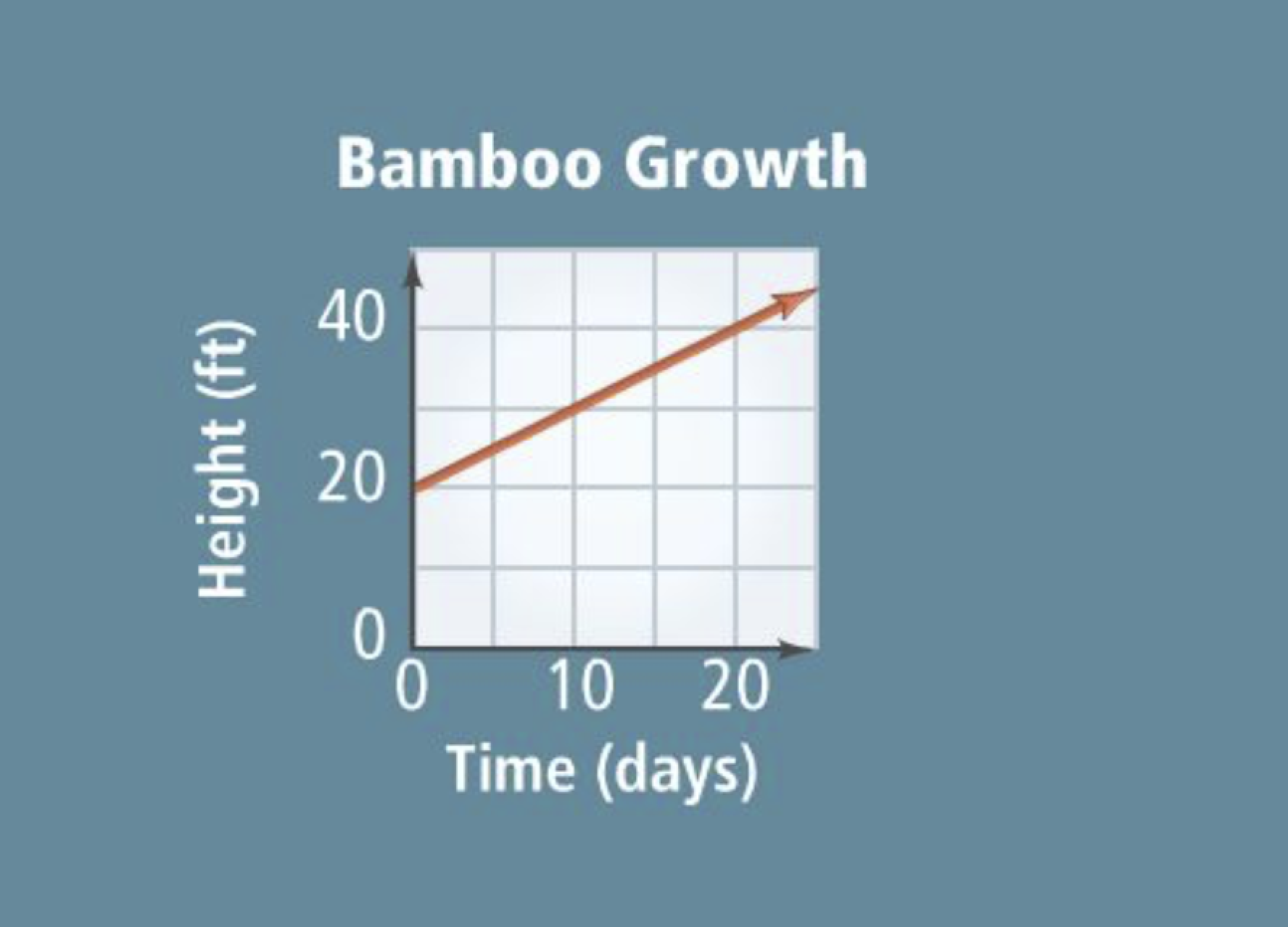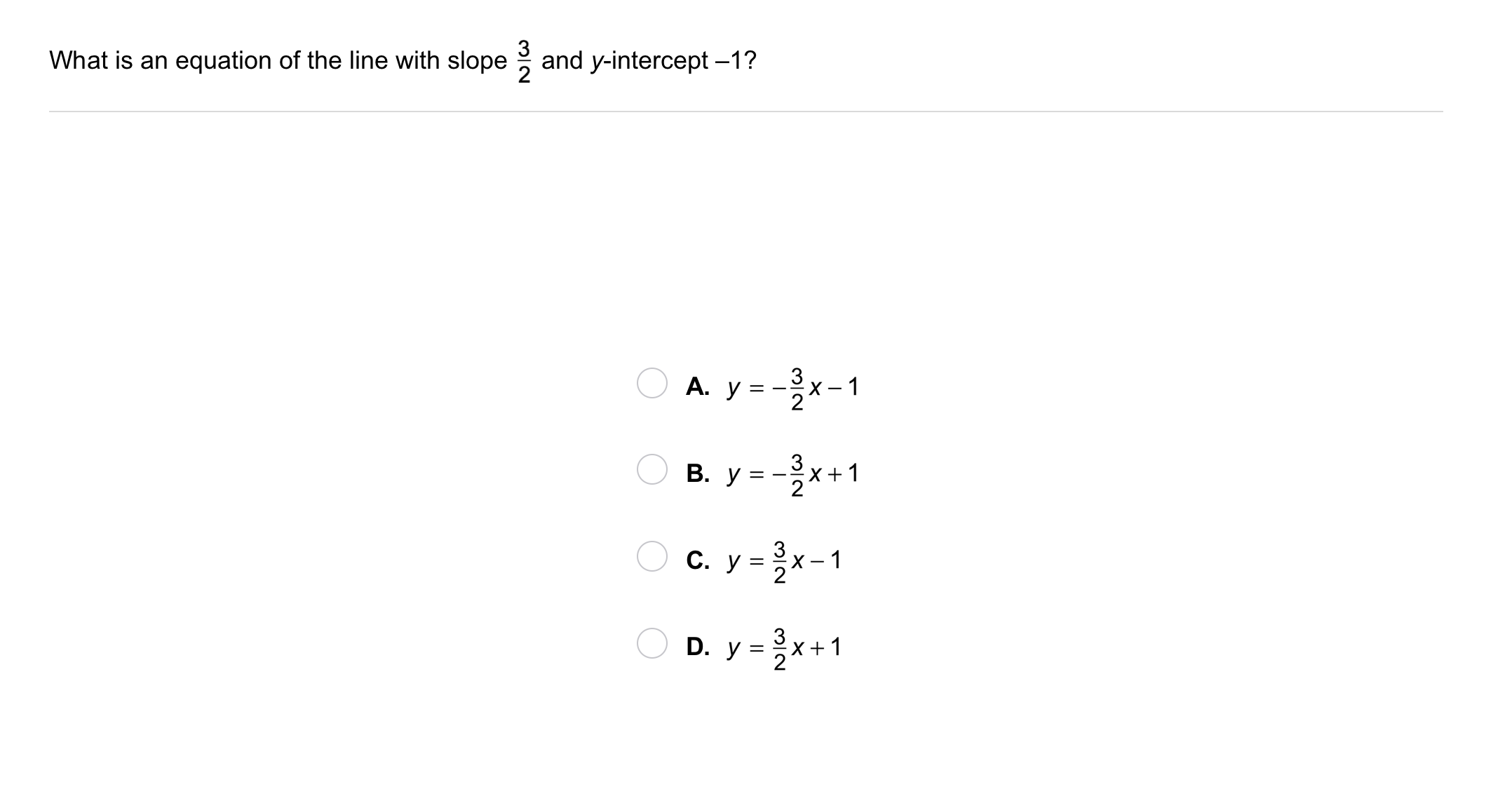Algebra 1 5-3 Complete Lesson: Slope-Intercept Form
starstarstarstarstarstarstarstarstarstar
5 (1 rating)
by Matthew Richardson
| 31 Questions
Note from the author:
A complete formative lesson with embedded slideshow, mini lecture screencasts, checks for understanding, practice items, mixed review, and reflection. I create these assignments to supplement each lesson of Pearson's Common Core Edition Algebra 1, Algebra 2, and Geometry courses. See also mathquest.net and twitter.com/mathquestEDU.The outlined content above was added from outside of Formative.1
2
1
10 pts
Solve It! Bamboo can grow very quickly. The graph models the growth of a bamboo plant. Find the point where the line crosses the vertical axis. What does this point tell you about the bamboo plant?
2
10 pts
Solve It! Find the slope of the line. What does the slope tell you about the bamboo plant?3
10 pts
Problem 1 Got It? What are the slope and y -intercept of the graph of the equation?

• -⅔
• ½
• Slope
• y-intercept
4
10 pts
Graphing: Graph the equation. Zoom and pan your graph to establish an appropriate viewing window.

5
10 pts
Problem 1 Got It? Reasoning: How do the graph of the line and the equation of the line in the previous item change if the y-intercept is moved down 3 units?6
6
10 pts
Problem 2 Got It?
A
B
C
D7
8
9
7
10 pts
Problem 3 Got It? What do you expect the slope of the line to be from looking at the graph?
negative
0
undefined
positive
8
10 pts
Problem 3 Got It? What is an equation of the line shown in the graph?
y = -x + 2
y= 2x + 1
y = 2x - 1
y = -2x + 2
9
5 pts
Problem 3 Got It? Reasoning: Does the equation of the line depend on the points you use to find the slope?
Yes
No10
10
10 pts
Problem 4 Got It?
A
B
C
D
11
10 pts
Problem 5 Got It? Graph the linear equation.
Be sure to include relevant graph detail: label axes, indicate units on both axes, and use arrows to represent end behavior, as appropriate.
12
10 pts
Problem 5 Got It? Graph the linear equation.
Be sure to include relevant graph detail: label axes, indicate units on both axes, and use arrows to represent end behavior, as appropriate.
13
10 pts
Problem 6 Got It? A plumber charges a \$65 fee for a repair plus \$35 per hour. Write an equation to model the total cost y of a repair that takes x hours.

Enter your equation with spaces on each side of operation symbols and the equal sign, like this: y = 27x + 93
14
10 pts
Problem 6 Got It? A plumber charges a \$65 fee for a repair plus \$35 per hour. Construct a graph to model the total cost.

Be sure to include relevant graph detail: label axes, indicate units on both axes, and use arrows to represent end behavior, as appropriate.15
10 pts
What is an equation of the line with slope 6 and y-intercept -4?
y = 6x - 4
y = -6x + 4
y = 6x + 4
y = -6x - 4
16
10 pts
What equation in slope-intercept form represents the line that passes through the points (-3, 4) and (2, -1)?
y = -x + 1
y = x + 1
y = x - 1
y = -x - 1
17
10 pts
What is the graph of y = 5x + 2?

Be sure to include relevant graph detail: label axes, indicate units on both axes, and use arrows to represent end behavior, as appropriate.18
10 pts
Vocabulary: Is y = 5 a linear equation? Explain.
19
5 pts
Reasoning: Is it always, sometimes, or never true that an equation in slope-intercept form represents a direct variation?
Always
Sometimes
Never
20
10 pts
Reasoning: Explain your response above.
21
10 pts
Writing: Describe two different methods you can use to graph the equation y = 2x + 4. Which method do you prefer? Explain.22
10 pts
Review Lesson 5-2: Suppose y varies directly with x. Match the given values of x and y with the direct variation equations.
• y = 5 when x = 1
• y = 8 when x = 4
• y = 9 when x = 3
• y = 2x
• y = 3x
• y = 5x
23
10 pts
Review Lesson 2-2: Solve the equation on the canvas. Justify each step.

24
10 pts
Review Lesson 1-7: Simplify the expression.

x + 15
-3x + 15
-3x - 5
-3x - 8
25
10 pts
Review Lesson 1-7: Simplify the expression.
Write your answer with a space on each side of the operation symbol, like this: 2x + 10
26
10 pts
Review Lesson 1-7: Simplify the expression.
Write your answer with a space on each side of the operation symbol, like this: 2x + 1027
10 pts
Vocabulary Review: Which equation is NOT a linear equation?

A
B
C
D
28
10 pts
Vocabulary Review: Classify each statement as true or false.
• true
• false
• The graph of a linear equation is always a horizontal line.
• The graph of a linear equation is always a straight line.
• The graph of a linear equation may be shaped like a "U."29
10 pts
Use Your Vocabulary: Complete each sentence with the correct word from the list.
• intercept
• intercepted
• interception
• During a football game, the home team's quarterback threw an __?__.
• The y-coordinate of a point where a graph crosses the y-axis is a y-__?__.
• The teacher __?__ the message Charlie was passing to his friend.
30
100 pts
Notes: Take a clear picture or screenshot of your Cornell notes for this lesson. Upload it to the canvas. Zoom and pan as needed.

For a refresher on the Cornell note-taking system, click here.
31
10 pts
Reflection: Math Success
Add to my formatives list

Formative uses cookies to allow us to better understand how the site is used. By continuing to use this site, you consent to the Terms of Service and Privacy Policy.